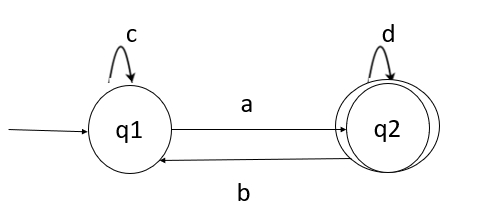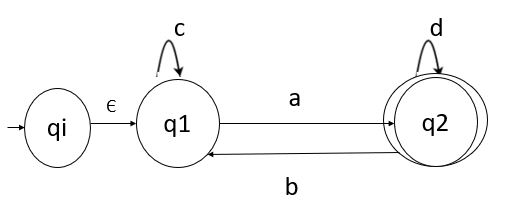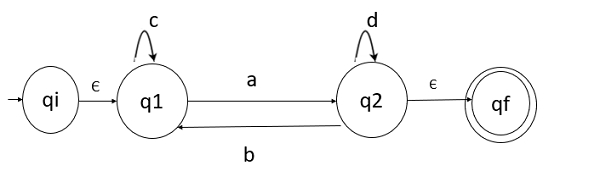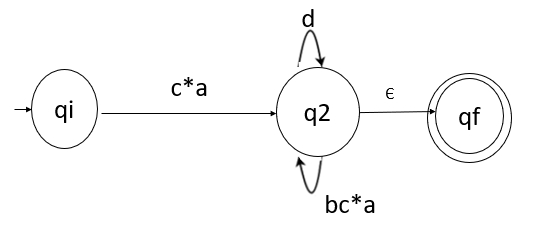# How to convert the finite automata into regular expressions?

## Problem

Convert the given finite automata (FA) into regular expression (RE).## Solution

There are two popular methods for converting a DFA to its regular expression −

• Arden’s Method
• State elimination method

Let’s consider the state elimination method to convert FA to RE.

### Rules

The rules for state elimination method are as follows −

### Rule 1

The initial state of DFA must not have any incoming edge.

If there is any incoming edge to the initial edge, then create a new initial state having no incoming edge to it.

### Rule 2

There must exist only one final state in DFA.

If there exist multiple final states, then convert all the final states into non-final states and create a new single final state.

### Rule 3

The final state of DFA must not have any outgoing edge.

If this exists, then create a new final state having no outgoing edge from it.

### Rule 4

Eliminate all intermediate states one by one.

Now, apply these rules to convert the FA to RE easily.

The given FA is as follows −### Step 1

Initial state q1 has an incoming edge so create a new initial state qi.### Step 2

Final state q2 has an outgoing edge. So, create a new final state qf.### Step 3

Start eliminating intermediate states

### First eliminate q1

There is a path going from qi to q2 via q1. So, after eliminating q1 we can connect a direct path from qi to q2 having cost.

εc*a=c*a

There is a loop on q2 using state qi. So, after eliminating q1 we put a direct loop to q2 having cost.

b.c*.a=bc*a

After eliminating q1, the FA looks like following −### Second eliminate q2

There is a direct path from qi to qf so, we can directly eliminate q2 having cost −

C*a(d+bc*a)* ε = c*a(d+bc*a)*

Which is our final regular expression for given finite automata.